### Home > A2C > Chapter Ch4 > Lesson 4.1.3 > Problem4-44

4-44.
1. Simplify each of the following expressions. Be sure that your answer has no negative or fractional exponents. Homework Help ✎

1. 641/3

2. (4x2 y5)2

3. (2x2 · y3)(3x1y 5)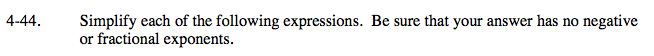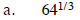What number, raised to the power of 3, is 64?

$\text{Another way to write it is }\sqrt{64}.$

4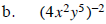Recall the Laws of Exponents.

$\text{Remember that }n^{-2}=\frac{1}{n^{2}}.$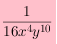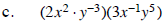$\left(2\cdot3\right)\left(x^{2}\cdot x^{-1}\right)\left(y^{-3}\cdot y^{5}\right)$